# CSAPP第三章读书笔记

CSAPP 第三章主要以理解 C 语言编译器产生的汇编代码为目的，简要介绍了 x86-64 汇编。

## 3.4 访问信息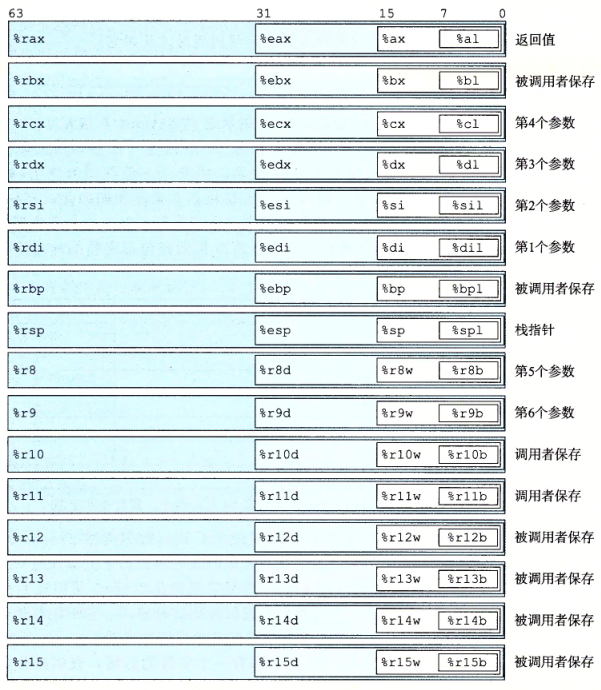## 3.5 算术和逻辑操作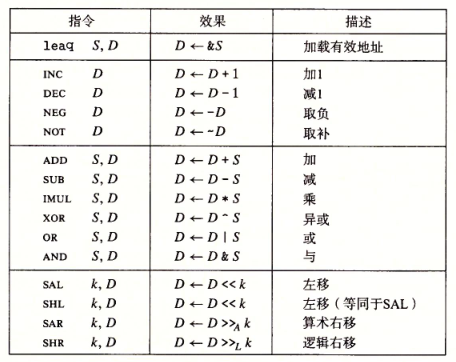### 3.5.1 加载有效地址

leaq （load effective address，加载有效地址）指令是 movq 指令的变体，用来将某个有效内存地址写入对应寄存器，作用类似 C 语言的取地址运算符 &。这条指令可以为后面的内存引用产生指针。leaq 的目的操作数只能是寄存器。

 1 2 3 4  long scale(long x, long y, long z) { long t = x + 4 * y + 12 * z; return t; } 
 1 2 3 4 5  scale: leaq (%rdi, %rsi, 4), %rax # x + 4 * y leaq (%rdx, %rdx, 2), %rdx # z + 2 * z = 3 * z leaq (%rax, %rdx, 4), %rax # (x + 4 * y) + 4 * (3 * z) = x + 4 * y + 12 * z ret 

${\bf N{\scriptsize OTE}}.$ 注意 GNU assembler 使用 # 处理单行注释。

#### 练习题 3.6

leaq 6(%rax), %rdx$x + 6$
leaq (%rax, %rcx), %rdx$x + y$
leaq (%rax, %rcx, 4), %rdx$x + 4y$
leaq 7(%rax, %rax, 8), %rdx$7 + 9x$
leaq 0xA(, %rcx, 4), %rdx${\tt 0xA} + 4y$
leaq 9(%rax, %rcx, 2), %rdx$9 + x + 2y$

#### 练习题 3.7

5 * x + 2 * y + 8 * z

### 3.5.2 一元和二元操作

#### 练习题 3.8

addq %rcx, (%rax)${\tt 0x100}$${\tt 0x100} subq %rdx, 8(%rax){\tt 0x108}$${\tt 0xA8}$
imulq $16, (%rax, %rdx, 8)${\tt 0x118}$${\tt 0x110} incq 16(%rax){\tt 0x110}$${\tt 0x14}$decq %rcx%rcx${\tt 0x0}$subq %rdx, %rax%rax${\tt 0xFD}$### 3.5.3 移位操作 移位指令先给出移位量，再给出要移位的量。其中，移位量可以是一个立即数或单字节寄存器 %cl（不能是其他寄存器），目的操作数可以是一个寄存器或内存位置。 当移位量为 %cl 时，具体的移位量由 %cl 的低$m$位决定：当移位操作对$w$位的数据进行操作时，$m$通过$2^m=w$来确定。 例如：当 %cl 的值为${\tt 0xFF = 0b1111,1111}$时，根据使用的指令不同，分别有： • 使用 salb 指令时，操作数据长度为 8 位，此时有$m = 3$，即位移量为低 3 位${\tt 0b111 = 7}$。 • 使用 salw 指令时，操作数据长度为 16 位，此时有$m = 4$，即位移量为低 4 位${\tt 0b1111 = 15}$。 • 使用 sall 指令时，操作数据长度为 32 位，此时有$m = 5$，即位移量为低 5 位${\tt 0b0001,1111 = 31}$。 • 使用 salq 指令时，操作数据长度为 64 位，此时有$m = 6$，即位移量为低 6 位${\tt 0b0011,1111 = 63}$。 移位指令中，salshlsarshr 指令分别代表算术左移逻辑左移算术右移逻辑右移，其中算术左移与逻辑左移的效果是相同的；算术右移的效果为用符号位填充空位，逻辑右移的效果为用 0 填充空位。 #### 练习题 3.9 salq$4, %rax

sarq %cl, %rax

### 3.5.4 讨论

 1 2 3 4 5 6 7  long arith(long x, long y, long z) { long t1 = x ^ y; long t2 = z * 48; long t3 = t1 & 0x0F0F0F0F; long t4 = t2 - t3; return t4; } 

 1 2 3 4 5 6 7  arith: xorq %rsi, %rdi # t1 = x ^ y leaq (%rdx, %rdx, 2), %rax # z + 2 * z = 3 * z salq $4, %rax # t2 = (3 * z) << 4 = 16 * (3 * z) = 48 * z andl$252645135, %edi # t3 = 0x0F0F0F0F & (x ^ y) subq %rdi, %rax # t2 - t3 ret 

• 使用了两条 leaq 和移位指令来计算小的乘法。
• 因为 0x0F0F0F0F 是一个双字长，因此使用 andl%edi 参与运算。

#### 练习题 3.10

 1 2 3 4 5 6 7  long arith2(long x, long y, long z) { long t1 = x | y; long t2 = t1 >> 3; long t3 = ~t2; long t4 = z - t3; return t4; } 

#### 练习题 3.11

A. 将 %rdx 清零。这是因为对任何 $x$，有 ${\rm XOR}(x,x) = 0$。

B. movq $0, %rdx C. 在 WSL(Ubuntu 20.04, Intel i5-3470) 平台上使用如下汇编代码编译目标文件：  1 2 3  a: xorq %rdx, %rdx b: movq$0, %rdx c: nop 

file.o:     file format elf64-x86-64

Disassembly of section .text:

0000000000000000 <a>:
0:   48 31 d2                xor    %rdx,%rdx

0000000000000003 <b>:
3:   48 c7 c2 00 00 00 00    mov    $0x0,%rdx 000000000000000a <c>: a: 90 nop  可见两条指令编码长度分别为 3 字节和 7 字节。${\bf N{\scriptsize OTE}}.$此外，通过使用「生成 4 字节值并以寄存器作为目的操作数的指令会将高 4 字节置为 0」的特性，可以使用 xorlmovl 指令进一步压缩长度。 如：将如下汇编代码编译为目标文件：  1 2 3  a: movl$0, %eax b: xorl %eax, %eax c: nop 

test.o:     file format elf64-x86-64

Disassembly of section .text:

0000000000000000 <a>:
0:   b8 00 00 00 00          mov    $0x0,%eax 0000000000000005 <b>: 5: 31 c0 xor %eax,%eax 0000000000000007 <c>: 7: 90 nop  可见 xorlmovl 指令只分别需要 2 字节和 5 字节。 关于「生成 4 字节值并以寄存器作为目的操作数的指令会将高 4 字节置为 0」这一特性的效果，可以使用 gdb 进行测试：先编译如下代码：  1 2 3 4 5 6 7 8  .section .text .globl _start _start: movq$0xABCDABCDABCDABCD, %rax xorl %eax, %eax movq $3, %rax xorl %eax, %eax nop  使用如下指令进行编译（--gstabs+ 参数用于保留符号信息，用于调试）：  1 2  as -o test.o test.s --gstabs+ ld -o test test.o  使用 gdb 进行调试，在 _start 处加断点，并使用 i register <寄存器名称> 打印寄存器的值： Copyright (C) 2020 Free Software Foundation, Inc. License GPLv3+: GNU GPL version 3 or later <http://gnu.org/licenses/gpl.html> This is free software: you are free to change and redistribute it. There is NO WARRANTY, to the extent permitted by law. Type "show copying" and "show warranty" for details. This GDB was configured as "x86_64-linux-gnu". Type "show configuration" for configuration details. For bug reporting instructions, please see: <http://www.gnu.org/software/gdb/bugs/>. Find the GDB manual and other documentation resources online at: <http://www.gnu.org/software/gdb/documentation/>. For help, type "help". Type "apropos word" to search for commands related to "word"... Reading symbols from testtest... (gdb) b _start Breakpoint 1 at 0x401000: file testtest.s, line 4. (gdb) run Starting program: /mnt/d/programming/tempanswertest/testtest Breakpoint 1, _start () at testtest.s:4 4 movq$0xABCDABCDABCDABCD, %rax
(gdb) i registers rax
rax            0x0                 0
(gdb) s
5           xorl %eax, %eax
(gdb) i registers rax
rax            0xabcdabcdabcdabcd  -6067004223159161907
(gdb) s
6           movq $3, %rax (gdb) i registers rax rax 0x0 0 (gdb) s 7 xorl %eax, %eax (gdb) i registers rax rax 0x3 3 (gdb) s 8 nop (gdb) i registers rax rax 0x0 0 (gdb) s Warning: Cannot insert breakpoint 0. Cannot access memory at address 0x1 0x0000000000401016 in ?? ()  ### 3.5.5 特殊的算术操作 考虑两个$w$位无符号整数$x,y$（$0 \leq x,y \leq 2^w - 1$），则有$0 \leq x \cdot y \leq (2^w - 1)^2 = 2^{2w}-2^{w+1}+1$，也即$x \cdot y$最多要用$2w$位来表示； 同样地，考虑两个$w$位有符号整数$x, y$（$-2^{w-1} \leq x,y \leq 2^{w-1}-1$），则有 $$-2^{w-1} \cdot (2^{w-1}-1) \leq x \cdot y \leq (-2^{w-1})^2$$ 即 $$-2^{2w-2}+2^{w-1} \leq x \cdot y \leq 2^{2w-2}$$ 最多需要$2w-2$位；但是，考虑到计算机内寄存器的数位长度通常以 2 为倍数进行倍增，可以认为无论有/无符号，$x \cdot y$均需最多$2w$位进行表示。 因此，x86-64 指令集对长度为 128 位的八字（oct word）提供了有限支持。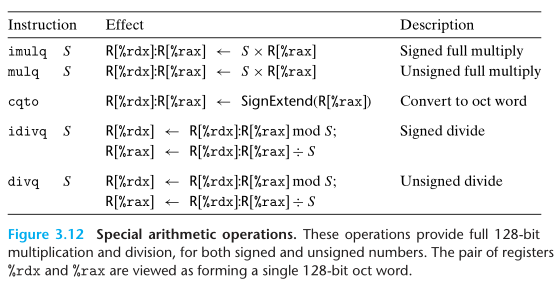在这组指令中，%rdx%rax 共同组成一个 128 位的八字。 在这组指令中，有两条单操作数乘法指令 imulqmulq，都要求将一个参数预存在 %rax 中，并将乘积结果的低 64 位放在 %rax 中，将高 64 位放在 %rdx 中。同时，x86-64 指令集还提供一个双操作数乘法指令 imulq（与单操作数乘法指令名称相同），如图 3-10 所示；但运算结果只截取低 64 位，和 C 语言的无符号/补码乘法运算相同（参考 2.3）。在只截取低 64 位的情况下，无符号/乘法运算结果的二进制位表示是相同的，因此只需要一条指令即可处理。${\bf N{\scriptsize OTE}}.$证明：$w$位的无符号乘法和补码乘法结果的二进制位表示相同。 考虑对位向量$\vec{x} = [x_{w-1},x_{w-2},\cdots,x_0],,,x_i \in {0,1},,,i=0,1,\cdots,w-1$，$\vec{x}$对应的无符号编码的值表示为 $$x_u = \sum_{i=0}^{w-1}x_i2^i$$$\vec{x}$对应的补码编码的值表示为 $$x_t = -x_{w-1}2^{w-1}+\sum_{i=0}^{w-2}x_i2^i$$ 显然有 $$x_u = x_t + x_{w-1}2^w$$ 因此，设$x,y$是$w$位无符号整数，则其对应的补码编码的值分别为$x’ = x + x_{w-1}2^w$，$y’ = y + y_{w-1}2^w$。此时，考虑$x’$与$y’$的乘积截取低$w\$ 位的结果得：

\newcommand{\modop}{,{\rm mod},} \begin{aligned} (x’ \cdot y’)\modop2^w &= [(x + x_{w-1}2^w) \cdot (y + y_{w-1}2^w)] \modop 2^w \ &= [(x \cdot y) + x \cdot y_{w-1}2^w + y \cdot x_{w-1}2^w + x_{w-1}y_{w-1}2^{2w}] \modop 2^w \ &= (x \cdot y) \modop 2^w \end{aligned}

#### 练习题 3.12

 1 2 3 4 5 6 7 8  uremdiv: movq %rdx, %r8 movq %rdi, %rax xorq %rdx, %rdx divq %rsi movq %rax, (%r8) movq %rdx, (%rcx) ret 

## 3.6 控制

### 3.6.1 条件码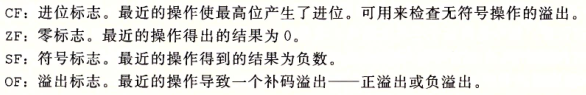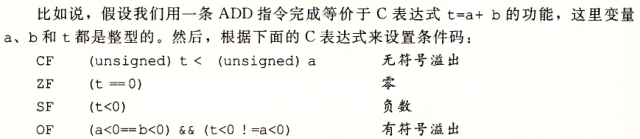• 逻辑操作，例如 xor，会将 CFOF 设置为 0。
• 移位操作会将 CF 设置为最后一个被移出的位，OF 设置为 0。
• incdec 指令会设置 OFZF 标志，但是不会改变 CF 标志。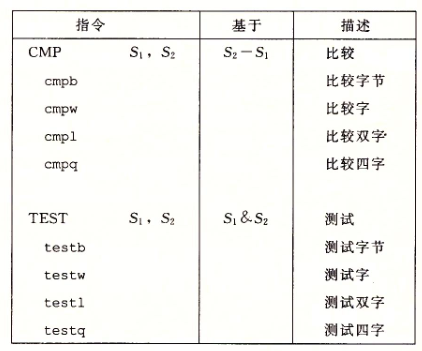test 指令的两个操作数相同时，可以用来判断一个值是负数，0，还是正数（例如，对 %rax 使用 testq %rax, %rax）；test 指令也可配合一个掩码来指定测试一个值中的某些位。